# JP6146197B2 - Design support method, design support program, and design support apparatus - Google Patents

Design support method, design support program, and design support apparatus Download PDF

## Info

Publication number
JP6146197B2
JP6146197B2 JP2013164627A JP2013164627A JP6146197B2 JP 6146197 B2 JP6146197 B2 JP 6146197B2 JP 2013164627 A JP2013164627 A JP 2013164627A JP 2013164627 A JP2013164627 A JP 2013164627A JP 6146197 B2 JP6146197 B2 JP 6146197B2
Authority
JP
Japan
Prior art keywords
program
support
value
command statement
executed
Prior art date
Legal status (The legal status is an assumption and is not a legal conclusion. Google has not performed a legal analysis and makes no representation as to the accuracy of the status listed.)
Active
Application number
JP2013164627A
Other languages
Japanese (ja)
Other versions
JP2015035033A (en
Inventor

Original Assignee

Priority date (The priority date is an assumption and is not a legal conclusion. Google has not performed a legal analysis and makes no representation as to the accuracy of the date listed.)
Filing date
Publication date
Application filed by 株式会社ソシオネクスト filed Critical 株式会社ソシオネクスト
Priority to JP2013164627A priority Critical patent/JP6146197B2/en
Publication of JP2015035033A publication Critical patent/JP2015035033A/en
Application granted granted Critical
Publication of JP6146197B2 publication Critical patent/JP6146197B2/en
Active legal-status Critical Current
Anticipated expiration legal-status Critical

• 238000000034 methods Methods 0.000 claims description 33
• 238000004364 calculation methods Methods 0.000 claims description 28
• 230000000875 corresponding Effects 0.000 claims description 19
• 238000009795 derivation Methods 0.000 claims description 4
• 238000010586 diagrams Methods 0.000 description 34
• 238000005457 optimization Methods 0.000 description 15
• 238000004458 analytical methods Methods 0.000 description 9
• 281000083854 First Command Financial Planning companies 0.000 description 8
• 239000004065 semiconductors Substances 0.000 description 8
• 238000003860 storage Methods 0.000 description 8
• 230000000576 supplementary Effects 0.000 description 8
• 230000015572 biosynthetic process Effects 0.000 description 5
• 238000004088 simulation Methods 0.000 description 5
• 230000003068 static Effects 0.000 description 5
• 238000003786 synthesis reactions Methods 0.000 description 5
• 230000002194 synthesizing Effects 0.000 description 5
• 238000004422 calculation algorithm Methods 0.000 description 4
• 238000006243 chemical reactions Methods 0.000 description 3
• 238000004519 manufacturing process Methods 0.000 description 2
• 230000003287 optical Effects 0.000 description 2
• 238000004891 communication Methods 0.000 description 1
• 238000005516 engineering processes Methods 0.000 description 1
• 238000006467 substitution reactions Methods 0.000 description 1

## Images

•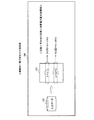•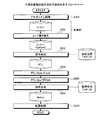••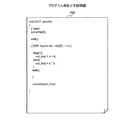•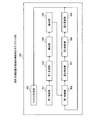•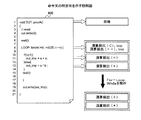•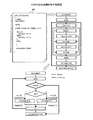•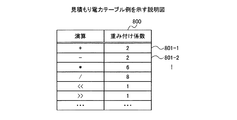•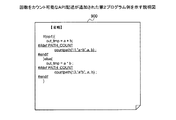••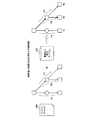•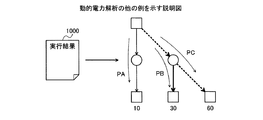••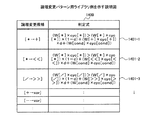•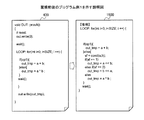•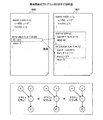•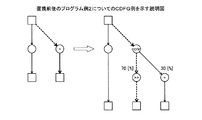••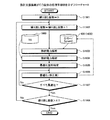••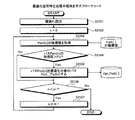•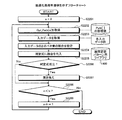## Description

The present invention relates to a design support method, a design support program, and a design support apparatus.

Conventionally, there is a technology for determining whether to implement each function in hardware or software based on the result of estimating the amount of processing and power consumption of each function defined in the program by the program parsing or instruction set simulator It is publicly known (for example, refer to Patent Document 1 below).

Conventionally, a technique for determining an instruction to be executed by an arithmetic unit mounted in an FPGA (Field Programmable Gate Array) based on the appearance frequency of each instruction in a program in which the operation of the system is described (for example, (See Patent Document 2 below.)

Also, a technique for assigning circuit elements to a program based on dynamic analysis data including either the number of variable data substitutions of points assigned to registers based on a data flow graph generated by the program or the number of times of holding data transition is known. (For example, refer to Patent Document 3 below.)

JP 2001-142927 A JP 2003-241975 A JP 2009-157440 A

However, in the high-level design, there is a problem that the design efficiency is low because the power consumption of each arithmetic unit when using the design target circuit is unknown.

In one aspect, an object of the present invention is to provide a design support method, a design support program, and a design support apparatus that can improve design efficiency.

According to one aspect of the present invention, the program is executed with input data that can be input to the arithmetic circuit for each of the command statements that specify a specific type of arithmetic among the programs that simulate the operation of the arithmetic circuit to be designed. A design that derives the number of times the statement is executed and calculates a value obtained by multiplying the number of times derived for each of the statements by a value corresponding to the amount of power required for the specific type of operation A support method, a design support program, and a design support apparatus are proposed.

According to one embodiment of the present invention, design efficiency can be improved.

FIG. 1 is an explanatory diagram showing an operation example of the present invention. FIG. 2 is a flowchart illustrating an example of a design procedure for a semiconductor integrated circuit. FIG. 3 is a block diagram illustrating a hardware configuration example of the design support apparatus. FIG. 4 is an explanatory diagram showing a program example. FIG. 5 is a block diagram illustrating a functional configuration example of the design support apparatus. FIG. 6 is an explanatory diagram showing a specific example of a command statement. FIG. 7 is an explanatory diagram illustrating a generation example of a CDFG. FIG. 8 is an explanatory diagram illustrating an example of an estimated power table. FIG. 9 is an explanatory diagram showing a second program example to which an API description capable of counting the number of times is added. FIG. 10 is an explanatory diagram illustrating an example of the execution result. FIG. 11 is an explanatory diagram illustrating another example of static power analysis. FIG. 12 is an explanatory diagram illustrating another example of dynamic power analysis. FIG. 13 is an explanatory diagram showing a specific example of a power of 2. FIG. 14 is an explanatory diagram of an example of a logical change pattern library. FIG. 15 is an explanatory diagram of Program Example 1 before and after replacement. FIG. 16 is an explanatory diagram showing a program example 2 before and after replacement. FIG. 17 is an explanatory diagram showing a CDFG example for the program example 2 before and after replacement. FIG. 18 is an explanatory diagram showing an example of RTL for program example 2 before and after replacement. FIG. 19 is a flowchart illustrating an example of an overall processing procedure performed by the design support apparatus. FIG. 20 is a flowchart showing an example of a static and dynamic power analysis processing procedure. FIG. 21 is a flowchart illustrating an example of an optimization location specifying process procedure. FIG. 22 is a flowchart illustrating an exemplary optimization processing procedure.

Exemplary embodiments of a design support method, a design support program, and a design support apparatus according to the present invention will be described below in detail with reference to the accompanying drawings.

FIG. 1 is an explanatory diagram showing an operation example of the present invention. The design support apparatus 100 is a computer that supports the design of an arithmetic circuit to be designed. The arithmetic circuit to be designed here includes a circuit having an arithmetic function. Hereinafter, an arithmetic circuit to be designed is referred to as a target circuit.

When the design support apparatus 100 executes the program 101 by using the input data 102 that can be input to the arithmetic circuit for each of the command statements instructing a specific type of arithmetic among the programs 101 that simulate the operation of the arithmetic circuit to be designed. Derives the number of times the statement is executed. Examples of specific types of operations include four arithmetic operations and logical operations. In the example of FIG. 1, the specific type of operation is division “/” and multiplication “*”.

Next, the design support apparatus 100 calculates a value obtained by multiplying the number of derived times and a value corresponding to the amount of power required for a specific type of operation for each of the statements. Here, the multiplied value is referred to as a power consumption index value. Thereby, it is possible to estimate the power consumption when the target circuit is used.

FIG. 2 is a flowchart illustrating an example of a design procedure for a semiconductor integrated circuit. First, in designing a semiconductor integrated circuit, an algorithm representing the function of the circuit to be designed is developed (step S201). As a result, a program in which the algorithm is coded in the C language, the C ++ language, or the like can be obtained. Next, in the design of the semiconductor integrated circuit, the command statement in the program is replaced (step S202). There are restrictions on the arithmetic units to be implemented, and programs are replaced to comply with this restriction.

In designing a semiconductor integrated circuit, high-level synthesis is performed using a synthesis tool (step S203). Thereby, a program described in RTL (Register Transfer Level) using a hardware description language or the like is obtained.

Next, in designing the semiconductor integrated circuit, the syntax of the RTL description is checked (step S204), and the RTL simulation is performed (step S205). In designing a semiconductor integrated circuit, logic synthesis is performed (step S206). Thereby, a net list is obtained. In designing the semiconductor integrated circuit, placement and routing are performed based on the net list (step S207).

Power estimation is performed by simulation using a netlist after logic synthesis. Therefore, when a design change occurs, it takes time to perform RTL design and high-level design again. On the other hand, since the design support apparatus 100 according to the present embodiment can perform power estimation in step S201 and step S202, the design efficiency can be improved.

(Example of hardware configuration of design support apparatus 100)
FIG. 3 is a block diagram illustrating a hardware configuration example of the design support apparatus. In FIG. 3, the design support apparatus 100 includes a CPU (Central Processing Unit) 301, a ROM (Read Only Memory) 302, a RAM (Random Access Memory) 303, a disk drive 304, and a disk 305. Yes. The design support apparatus 100 includes an I / F 306, an input device 307, and an output device 308. Each unit is connected by a bus 300.

Here, the CPU 301 governs overall control of the design support apparatus 100. The ROM 302 stores a program such as a boot program. The RAM 303 is used as a work area for the CPU 301. The disk drive 304 controls reading / writing of data with respect to the disk 305 according to the control of the CPU 301. The disk 305 stores data written under the control of the disk drive 304. Examples of the disk 305 include a magnetic disk and an optical disk.

The I / F 306 is connected to a network NET such as a LAN (Local Area Network), a WAN (Wide Area Network), and the Internet through a communication line, and is connected to other devices via the network NET. The I / F 306 controls an internal interface with the network NET, and controls data input / output from an external device. For example, a modem or a LAN adapter can be used as the I / F 306.

The input device 307 is an interface for inputting various data by a user operation such as a keyboard, a mouse, and a touch panel. The input device 307 can also capture images and moving images from the camera. The input device 307 can also capture audio from a microphone. The output device 308 is an interface that outputs data according to an instruction from the CPU 301. Examples of the output device 308 include a display and a printer.

FIG. 4 is an explanatory diagram showing a program example. The first program 400 can simulate the operation of the target circuit and is described in C language, C ++ language, or the like.

(Functional configuration example of the design support apparatus 100)
FIG. 5 is a block diagram illustrating a functional configuration example of the design support apparatus. The design support apparatus 100 includes a first specification unit 501, a CDFG generation unit 502, a first generation unit 503, a derivation unit 504, a calculation unit 505, a first determination unit 506, a second specification unit 507, A second determination unit 508 and a second generation unit 509 are included. The processing of each unit is coded in a design support program stored in a storage device accessible by the CPU 301, for example. Then, the CPU 301 reads the design support program from the storage device and executes the process coded in the design support program. Thereby, the process of each part is implement | achieved. Further, the processing results of each unit are stored in a storage device such as the RAM 303 and the disk 305, for example.

First, the first specifying unit 501 specifies a command statement instructing to perform at least one of a plurality of types of operations from the first program 400 that simulates the operation of the target circuit. Examples of the plural types of operations include four arithmetic operations, logical operations, combinations of logical operations and four arithmetic operations. Specifically, the first specifying unit 501 scans the first program 400 line by line and specifies a command statement having an operation description.

FIG. 6 is an explanatory diagram showing a specific example of a command statement. The first specifying unit 501 specifies a command statement describing the comparison operation “<” on the eighth line from the first program 400. The first specifying unit 501 specifies a command statement in which addition “++” is described in the eighth line from the first program 400. The first specifying unit 501 specifies a command statement in which the addition “+” on the eleventh line is described from the first program 400. The first specifying unit 501 specifies the command statement in which the multiplication “*” in the 13th line is described from the first program 400.

In addition, the first specifying unit 501 excludes For-Loop statements and While statements from among the specified command statements. As a result, the addition “+” in the 11th row and the multiplication “*” in the 13th row are specified. Since the For-Loop statement and the While statement are optimized by the high-level synthesis tool, they are excluded from the processing targets in the present invention.

The CDFG generation unit 502 generates a CDFG (Control Data Flow Graph) based on the first program 400. CDFG is information describing a control flow and a data flow when the target circuit is mounted. Here, CDFG is information to be output via an output device 308 such as a display for easy understanding.

FIG. 7 is an explanatory diagram illustrating a generation example of a CDFG. The CDFG generation unit 502 generates a CDFG corresponding to the first program 400, for example. Here, according to the CDFG, the specified statements constitute a path in the CDFG. Therefore, the identification information indicating the specified command statement, the operation indicated by the command statement, and the argument of the operation are associated with each other and are hereinafter referred to as a path. Here, the instruction sentence indicated by the identification information included in the path is referred to as a path instruction sentence.

The first specifying unit 501 acquires a weighting coefficient from the estimated power table for each path. The weighting coefficient is a value corresponding to the power consumption value of the calculation according to the type of calculation.

FIG. 8 is an explanatory diagram illustrating an example of an estimated power table. The estimated power table 800 stores a weighting coefficient for each calculation. The estimated power table 800 has calculation and weighting coefficient fields. By setting information in each field, it is stored as a record (801-1 to 801-2, etc.). The estimated power table 800 is stored in a storage device such as the RAM 303 and the disk 305, for example.

Identification information indicating each calculation is set in the calculation field. In the weighting coefficient field, a value corresponding to the amount of power required for each calculation is set. Here, for example, the value corresponding to the power amount is set to the power consumption amount [W / Cycle] for each unit execution cycle. However, the value is not limited to this, and the power amount required for the calculation can be relatively specified. Also good.

The first generation unit 503 is a second program 900 that simulates the operation of the target circuit based on the first program 400, and includes a second program 900 that includes a command statement that instructs counting of the number of times for each path. Execute the process to generate. Here, the number of times is the number of times that the specified statement is executed when the first program 400 is executed with the input data. The input data here is information created by the user based on the design specifications of the target circuit. The input data is, for example, a signal value that can be input to the input terminal of the target circuit. Here, the operation of the target circuit is simulated by executing the second program 900 based on the input data. Information including input data and executing each program is referred to as a test bench.

FIG. 9 is an explanatory diagram showing a second program example to which an API description capable of counting the number of times is added. The second program 900 describes a countpath (number of lines, calculation formula, operand) which is an API (Application Program Interface) description that can count the number of times.

Next, the deriving unit 504 derives the number of times that the operation indicated by the specified statement is performed when the first program 400 is executed based on the input data. Specifically, the deriving unit 504 counts the number of times that the specified command statement is executed by executing simulation using the second program 900 in which the API is inserted and the test bench.

The calculation unit 505 requires an operation specified by the specified instruction when input data is input to the target circuit based on the derived number of times and a weighting coefficient corresponding to the operation specified by the specified instruction. An index value for the electric energy is calculated.

FIG. 10 is an explanatory diagram illustrating an example of the execution result. In the execution result 1000, the value of “i” and the value of “op1” are shown together for easy understanding. For example, the number of times the command statement of the path Path  is executed is 3, and the number of times the command statement of the Path  is executed is 2.

The calculating unit 505 calculates an index value obtained by multiplying the derived number by a value corresponding to the amount of power required for the operation indicated by the command statement. As described above, the value corresponding to the amount of power required for the calculation is a weighting coefficient. For example, the index value of each path is as follows.

Index value of path Path  = 2 × 3 = 6
Index value of path Path  = 6 × 2 = 12

FIG. 11 is an explanatory diagram illustrating another example of static power analysis. The first specifying unit 501 may specify the ratio of the weighting factor for each path to the total value of the weighting factors acquired for each path. The example program shown in FIG. 11 is different from the first program 400 described above. For easy understanding, a square in CDFG in FIG. 11 indicates FF (Flip Flop). In the example of FIG. 11, a relative ratio RP (relative power) is specified when the sum of the weighting coefficients is 100 [%]. Thus, the relative ratio RP of the path PA is 10, the relative ratio RP of the path PB is 50, and the relative ratio RP of the path PC is 40. Thereby, in the example of a figure, it is specified that the path | pass PB is the highest relative ratio RP.

FIG. 12 is an explanatory diagram illustrating another example of dynamic power analysis. In the example of FIG. 12, the calculation unit 505 calculates an index value obtained by multiplying the value according to the derived number of times by the value according to the amount of power required for the calculation. In the example of FIG. 12, the value corresponding to the derived number is the operation rate. Therefore, the total operation rate is 100 [%]. The operation rate of the path PA is 10 [%], the operation rate of the path PB is 30 [%], and the operation rate of the path PC is 60 [%].

For example, the index value of the path PA is 100, the index value of the path PB is 1500, and the index value of the path PC is 2400. As described above, regarding the relative ratio PR, the path PB is the largest, but since the pass PC is executed many times, the index value is the largest for the path PC.

Next, the first determination unit 506 determines whether or not the index value calculated for each identified path satisfies a first predetermined condition. Here, the first predetermined condition is determined in advance by the user and stored in a storage device such as the disk 305 or the RAM 303. The first predetermined condition is, for example, whether or not the index value is greater than or equal to a threshold value. For example, the first determination unit 506 determines whether or not the index value is greater than or equal to a threshold value. For example, the threshold is determined by the user and stored in a storage device such as the disk 305 or the RAM 303. Here, the threshold is expressed as PJ.

The second generation unit 509 generates a third program in which the command statement of the path for which the first determination unit 506 has determined that the index value is greater than or equal to the threshold value PJ. A path for which the index value is determined to satisfy the predetermined condition by the first determination unit 506 is output as an optimization target path. Here, for example, the threshold PJ is set to 10, and the path Path  is output as the optimization target path Opt_Path. The calculated index value, the arithmetic expression, the identification information of the command statement, and the argument given to the arithmetic expression are associated and stored as an optimization target path. The identification information of the command statement is, for example, the number of lines when counted from the top in the first program 400.

For example, the second generation unit 509 generates a third program based on the first program 400. The third program is, for example, a program in which a first command statement in which a calculated index value satisfies a first predetermined condition in a command statement is replaced with a command statement instructing an operation equivalent to a specific type of operation. Further, the third program sets the first imperative sentence as a second predetermined condition based on any one of the first imperative sentence and a second imperative sentence that instructs an operation equivalent to the operation indicated by the first imperative sentence. This is a program that is replaced with a command statement instructing execution in accordance with the above.

The third program may be generated after determining whether or not to replace the command statement. Therefore, when the equivalent operation is a shift operation, the second specifying unit 507 sets the operand value to a power of 2 among specific types of operations performed when the first program 400 is executed with input data. Identify the percentage of certain types of operations. Then, the second determination unit 508 determines whether or not the specified ratio satisfies the third predetermined condition.

Specifically, the second specifying unit 507 acquires the input data of the operand that is a combination of values that are the operands of the path statement in the simulation by executing the second program 900 with the input data. . Then, the second specifying unit 507 counts the number that is a power of 2 among the values that are operands.

FIG. 13 is an explanatory diagram showing a specific example of a power of 2. The input data 1300 of the operand is a combination of values input for each of a and b that are operands of the statement described in the eleventh line in the first program 400.

Table 1301 shows the power of 2 of the operand a, the power of 2 of the operand b, the number M of times that neither the operand a nor the operand b is a power of 2, and the operand a or operand. The number S of times when any of the operators b is a power of 2. The number of times M is finally 3 and the number of times S is finally 7.

Next, the second specifying unit 507 subtracts a logical change candidate corresponding to the calculation for the optimization target path from the logical change pattern library. Then, the second specifying unit 507 acquires a determination formula for the logical change candidate.

FIG. 14 is an explanatory diagram of an example of a logical change pattern library. The logic change pattern library 1400 includes fields for logic change candidates and determination formulas. By setting information in each field, it is stored as a record (1401-1 to 1401-3, etc.). The logical change pattern library 1400 is stored in a storage device such as the disk 305.

According to the logic change pattern library 1400, for example, multiplication “*” is replaced with addition “+” or shift operation “<<”, and division “/” is replaced with shift operation “>>”. Although not shown, the addition “+” is replaced with the exclusive OR “xor”. “−” Is replaced with “xor”.

Here, since the calculation of the path Path  is multiplication “*”, the second specifying unit 507 acquires a determination formula associated with the conversion candidate [* → <<], for example. For example, the second determination unit 508 determines whether or not the determination formula is satisfied by assigning a value to each argument of the determination formula. Each argument of the judgment formula is as follows. d is a ratio that is a power of 2, and (1-d) is a ratio that is not a power of 2. In the example of the conversion candidate [* → <<], d is the rate at which the shift operation “<<” is executed, and (1-d) is the rate at which the multiplication “×” is executed. W [] is a weighting coefficient for the calculation in []. As described above, for example, the unit of W [] is [W / Cycle]. cyc [] is the number of execution cycles of multiplication in []. The number of execution cycles for each type of operation is determined in advance and stored in a storage device such as the disk 305. Alternatively, the number of execution cycles may be previously substituted into the determination formula. The number of execution cycles for division is 16, but the number of execution cycles for other operations is 1. cond is a condition that is a power of 2, and is a second predetermined condition described later. W [cond] is a weighting coefficient of a logic circuit for condition determination (hereinafter referred to as “condition determination circuit”) that is a power of two. Here, when the condition determination circuit is a bit operation circuit or a subtraction circuit, the weighting coefficient is 2. cyc [cond] is the number of execution cycles of the condition determination circuit. When the condition determination circuit is a bit operation circuit, it is 1. When the condition determination circuit is a subtraction circuit, the subtraction “−” weighting coefficient stored in the estimated power table 800 is used. Specific examples in this embodiment will be shown below.

d = S / (S + M) = 7 / (7 + 3) = 0.7
1-d = 0.3
W [*] = 6
cyc [*] = 1
W [<<] = 1
cyc [<<] = 1
W [cond] = 2
cyc [cond] = 1

When the value of each argument is substituted into the determination formula, the second determination unit 508 holds the determination formula because the left side is 6 and the right side is 2.5. Therefore, when the determination formula holds, the second generation unit 509 generates the third program as described above. In addition, the third program includes a first command statement whose index value satisfies the first predetermined condition, a first command statement, and a second command statement that instructs an operation equivalent to the operation indicated by the first command statement. This is a program in which one of them is replaced with a command statement instructing execution according to the second predetermined condition.

For example, when the operation indicated by the statement that satisfies the first predetermined condition is multiplication and the equivalent operation is a shift operation, the second predetermined condition is that the value that becomes the operand in the multiplication is a power of 2 Whether or not. The instruction statement instructing execution according to the second predetermined condition indicates that the instruction statement instructing an equivalent operation is executed when the value to be the operand is a power of 2, and the operand When the value to be is not a power of 2, it is instructed to execute a statement that satisfies the first predetermined condition.

FIG. 15 is an explanatory diagram of Program Example 1 before and after replacement. The left side is the first program 400 before replacement, and the right side is the third program 1500 after replacement. For example, the third program 1500 includes a command statement that instructs a shift operation “<<” with a shift number as an operand a, and a command that instructs a shift operation “<<” with a shift number as an operand b. , And a command statement for instructing multiplication “*” are described. In the third program 1500, any command statement is executed according to the condition of the if statement. The condition of the “if” statement is determined by the result of the cond () function.

According to the cond () function, if it is a power of the value 2 of the operand a, an instruction for instructing a shift operation “<<” with the shift number as the value of the operand a is executed. According to the cond () function, if the value of the operand b is a power of 2, an instruction for instructing a shift operation “<<” using the shift number as the value of the operand b is executed. According to the cond () function, if neither of the operands a and b is a power of 2, an instruction for instructing multiplication “*” is executed.

Also, for example, when the operation indicated by the statement that satisfies the first predetermined condition is division and the equivalent operation is a shift operation, the second predetermined condition is that the value that is a divisor in the division is a power of 2 Whether or not. For example, a command statement instructing execution in accordance with the second predetermined condition instructs to execute a command statement instructing an equivalent operation when the value to be a divisor is a power of 2 and becomes a divisor. When the value is not a power of 2, it instructs to execute a statement that satisfies the first predetermined condition.

FIG. 16 is an explanatory diagram showing a program example 2 before and after replacement. The left side is the first program 1600 before replacement, and the right side is the third program 1601 after replacement. The third program 1601 describes an instruction for instructing a shift operation “<<” and an instruction for instructing a division “/” with the number of shifts as the operand Y, which is executed by the cond () function. It is decided whether to be done. The lower side of the figure shows the relationship between the first program 1600 and the third program 1601.

According to the cond () function, if the value of the operand Y that is the dividend is a power of 2, an instruction that instructs a shift operation “>>” that uses the shift number as the value of the operand Y is executed. . According to the cond () function, if b is a power of 2, an instruction that instructs a shift operation “>>” with the shift number as the value of the operand b is executed. According to the cond () function, if the value of the operand Y, which is the dividend, is not a power of 2, an instruction for instructing division “/” is executed.

FIG. 17 is an explanatory diagram showing a CDFG example for the program example 2 before and after replacement. FIG. 18 is an explanatory diagram showing an example of RTL for program example 2 before and after replacement. For example, in the first program 1600 before replacement, when the weighting coefficient of division “/” is 8 and the number of times is n, the index value of the power consumption is 8n. In the third program 1601 after replacement, for example, in the cond () function, the weighting coefficient of division “/” is 8, and the weighting coefficient of shift operation “>>” is 1, so division “/” is selected. If the probability is 30 [%], the index value is 3.1 n (= 0.7 × n × 1 + 0.3 × n × 8). In such a case, the power consumption can be reduced nearly twice.

(Optimization processing procedure performed by the design support apparatus 100)
FIG. 19 is a flowchart illustrating an example of an overall processing procedure performed by the design support apparatus. In the present embodiment, the processing procedure performed by the design support apparatus 100 will be described by dividing it into three phases from the first phase to the third phase.

The design support apparatus 100 sets the number of repetitions = 0 (step S1901). The design support apparatus 100 sets the number of repetitions = the number of repetitions + 1 (step S1902). The design support apparatus 100 performs static power analysis based on the first program 400 (step S1903). Next, the design support apparatus 100 performs dynamic power analysis by executing the first program 400 using the test bench 1900 (step S1904). As described above, the test bench 1900 includes the input data 102 that can be input to the target circuit. The design support apparatus 100 identifies an optimization location based on the simulation result (step S1905).

The design support apparatus 100 optimizes the identified optimization location (step S1906). The design support apparatus 100 determines whether all have been optimized (step S1907). If it is determined that the optimization has not been completed (step S1907: NO), the design support apparatus 100 returns to step S1906. If it is determined that all have been optimized (step S1907: YES), the design support apparatus 100 determines whether the number of iterations is greater than a predetermined number X (step S1908). The predetermined number X is stored in advance in the disk 305, the RAM 303, or the like.

If the number of repetitions is equal to or less than the predetermined number X (step S1908: No), the design support apparatus 100 returns to step S1902. As a result, the optimized program can be optimized again. If the number of repetitions is greater than the predetermined number X (step S1908: Yes), the design support apparatus 100 ends a series of processes. In this way, the optimized program can be retargeted again until the predetermined number X is reached.

FIG. 20 is a flowchart showing an example of a static and dynamic power analysis processing procedure. The design support apparatus 100 acquires a program (step S2001). Next, the design support apparatus 100 sets n = 0 (step S2002). The design support apparatus 100 determines whether there is an unselected command statement (step S2003). When there is an unselected command statement (step S2003: Yes), the design support apparatus 100 selects the first command statement from the unselected command statements (step S2004). Next, the design support apparatus 100 determines whether or not the selected command statement is a command statement instructing a specific type of operation (step S2005). If the selected command statement is not a command statement for instructing a specific type of operation (step S2005: No), the design support apparatus 100 returns to step S2003.

If the selected command statement is a command statement that instructs a specific type of operation (step S2005: Yes), the design support apparatus 100 determines whether the selected command statement is a For-Loop statement or a While statement. (Step S2006). If the selected command statement is either a For-Loop statement or a While statement (step S2006: Yes), the design support apparatus 100 returns to step S2003.

When the selected command statement is neither a For-Loop statement nor a While statement (step S2006: No), the design support apparatus 100 associates the identification information of the selected command statement, the operation content, and the operand, and passes the path Path. [N] is set (step S2007). The design support apparatus 100 sets n = n + 1 (step S2008). The design support apparatus 100 adds a counting API description to the program (step S2009), and returns to step S2003. In step S2009, the above-described second program 900 is obtained.

If there is no unselected command statement (step S2003: No), the design support apparatus 100 executes the program using the test bench (step S2010). The design support apparatus 100 calculates an index value for each path Path [0 to n] based on the number of times and the weighting coefficient for the calculation of the path Path (step S2011). The design support apparatus 100 outputs the calculation result (step S2012) and ends the series of processes.

FIG. 21 is a flowchart illustrating an example of an optimization location specifying process procedure. The design support apparatus 100 sets a threshold value PJ by a user operation (step S2101). Next, the design support apparatus 100 sets n = 0 (step S2102). The design support apparatus 100 acquires an index value of the path Path [n] (step S2103). The design support apparatus 100 determines whether or not the index value of the path Path [n]> the threshold value PJ (step S2104).

When the index value of the path Path [n] is not greater than the threshold value PJ (step S2104: No), the process proceeds to step S2106. When the index value of the path Path [n]> the threshold value PJ (step S2104: Yes), the design support apparatus 100 sets the path Path [n] as the optimization target path Opt_Path (step S2105). The design support apparatus 100 determines whether n <the number m of paths (step S2106). If n <the number m of paths (step S2106: Yes), the design support apparatus 100 sets n + = 1 (step S2107) and returns to step S2103. If n <the number m of paths is not satisfied (step S2106: No), the design support apparatus 100 ends a series of processes.

FIG. 22 is a flowchart illustrating an exemplary optimization processing procedure. First, the design support apparatus 100 sets n = 0 (step S2201). Next, the design support apparatus 100 acquires the optimization target path Opt_Path [n] (step S2202). The design support apparatus 100 acquires input data that is the value of the operand of the path Opt_Path [n] (step S2203).

The design support apparatus 100 specifies the power-of-two ratio of the input data (step S2204). The design support apparatus 100 substitutes the ratio specified in the determination formula from the logic change pattern library 1400 (step S2205). The design support apparatus 100 determines whether or not the determination formula is satisfied (step S2206). If the determination formula is not satisfied (step S2206: NO), the design support apparatus 100 proceeds to step S2208.

When the determination formula is satisfied (step S2206: Yes), the design support apparatus 100 replaces the command statement in the path Opt_Path [n] with a conversion candidate (step S2207). The design support apparatus 100 determines whether or not n <the number k of optimization target paths (step S2208). When n <the number k of paths to be optimized (step S2208: Yes), the design support apparatus 100 sets n + = 1 (step S2209) and returns to step S2202.

If n <the number of paths to be optimized is not k (step S2208: No), the design support apparatus 100 ends a series of processes.

As described above, the design support apparatus 100 according to the present embodiment, for each command statement instructing a specific type of operation in the program that simulates the operation of the target circuit, the command statement in the input data of the target circuit. An index value of the power consumption corresponding to the number of executions of is calculated. This makes it possible to estimate the amount of power consumed when the target circuit is used in high-level design. Therefore, design efficiency can be improved.

In addition, the design support apparatus 100 replaces a command statement in which the calculated index value satisfies the first predetermined condition in the command statement with a command statement instructing an operation equivalent to the operation instructed by the command statement satisfying the predetermined condition. Is generated. Thereby, the power consumption can be reduced by changing the algorithm.

In addition, the design support apparatus 100 identifies the first command statement in which the calculated index value satisfies the first predetermined condition among the command statements. Then, the design support apparatus 100 executes either the first command statement or the second command statement instructing an operation equivalent to the operation instructed by the first command statement according to the second predetermined condition. The second program is generated by replacing the command statement. Thus, the power consumption can be reduced by changing the algorithm so that any one of the plurality of instructions can be executed.

Further, when the operation is division and the equivalent operation is a shift operation, the design support apparatus 100 executes the second command statement when the value of the dividend is a power of 2, and performs the second operation when the value is not a power of 2. A second program replaced with a command statement instructing execution of one command statement is generated. As a result, if the dividend is a power of 2, a shift operation with less power consumption than division is possible, so that low power consumption can be achieved.

When the operation is multiplication and the equivalent operation is shift operation, the design support apparatus 100 executes the second command statement when the operand value is a power of 2, and the design support apparatus 100 is not a power of 2. A second program is generated in which the first instruction is replaced with an instruction that instructs execution of the first instruction. As a result, if the operand is a power of 2, a shift operation with less power consumption than multiplication is possible, so that power consumption can be reduced.

In addition, the design support apparatus 100 is a program in which a command statement is replaced by a ratio of a specific type of operation whose operand value is a power of 2 among specific types of operations performed when the program is executed with input data. Determine whether to generate. As a result, it is possible to determine whether the power consumption is reduced by replacing the command statement.

In addition, the design support apparatus 100 generates a program including a command statement that instructs counting of the number of times of execution for each command statement that specifies a specific type of operation. As a result, it is possible to derive the number of times that a command statement instructing a specific type of operation is executed only by executing the program.

The design support method described in this embodiment can be realized by executing a design support program prepared in advance on a computer such as a personal computer or a workstation. The design support program is recorded on a computer-readable recording medium such as a magnetic disk, an optical disk, or a USB (Universal Serial Bus) flash memory, and is executed by being read from the recording medium by the computer. The design support program may be distributed through a network such as the Internet.

The following additional notes are disclosed with respect to the embodiment described above.

(Supplementary note 1)
For each command statement that designates a specific type of operation in a program that simulates the operation of the design target arithmetic circuit, the command statement is executed when the program is executed with input data that can be input to the arithmetic circuit. Derived the number of times
For each of the statements, calculate a value obtained by multiplying the derived number of times by a value corresponding to the amount of power required for the specific type of operation.
A design support method characterized by executing processing.

(Appendix 2) The computer
Executing a process of generating a program in which a command statement in which the calculated value satisfies a predetermined condition in the command statement is replaced with a command statement instructing an operation equivalent to an operation instructed by the command statement satisfying the predetermined condition The design support method according to appendix 1, characterized by:

(Supplementary note 3)
Based on the program (hereinafter, referred to as “first program”), a statement that satisfies the first predetermined condition in which the calculated value among the statements satisfies the first predetermined condition, and the first statement that satisfies the first predetermined condition, A second program in which any one of a command statement instructing an operation equivalent to a calculation instructed by a command statement satisfying a predetermined condition is replaced with a command statement instructing execution according to a second predetermined condition The design support method according to appendix 1, wherein the process of generating is executed.

(Supplementary Note 4) In the case where the operation indicated by the statement that satisfies the first predetermined condition is division and the equivalent operation is a shift operation,
The second predetermined condition is whether or not a value to be a divisor in the division is a power of 2,
The command statement instructing execution according to the second predetermined condition indicates that the command statement instructing the equivalent operation is executed when the value to be the divisor is a power of 2, and the divisor 4. The design support method according to appendix 3, wherein an instruction to execute a statement that satisfies the first predetermined condition is given when the value to be is not a power of 2.

(Supplementary Note 5) In the case where the operation indicated by the statement that satisfies the first predetermined condition is multiplication and the equivalent operation is a shift operation,
The second predetermined condition is whether or not a value to be an operand in the multiplication is a power of 2,
The command statement instructing execution according to the second predetermined condition instructs to execute the command statement instructing the equivalent operation when the value to be the operand is a power of two, The design support method according to appendix 3, wherein an instruction to execute a statement that satisfies the first predetermined condition is executed when the value to be the operand is not a power of 2.

(Supplementary Note 6) In the case where the equivalent operation is a shift operation,
The computer is
Executing a process of specifying a ratio of the specific type of operation whose value to be an operand is a power of 2 among the specific type of operation performed when the first program is executed by the input data;
In the process of generating the second program, the second program is not generated when the specified ratio does not satisfy the third predetermined condition. The design support according to any one of appendices 3 to 5, Method.

(Supplementary note 7)
From the program (hereinafter referred to as the “first program”), a command statement for instructing the specific type of operation is specified,
A process of generating a second program that simulates the operation of the arithmetic circuit based on the first program and includes a command statement that instructs the counting of the number of times for each of the specified command statements Run
In the process of deriving the number of times, the number of times is derived for each of the specified statements by executing the second program with the input data. The design support method described.

(Appendix 8)
For each command statement that designates a specific type of operation in a program that simulates the operation of the design target arithmetic circuit, the command statement is executed when the program is executed with input data that can be input to the arithmetic circuit. Derived the number of times
For each of the statements, calculate a value obtained by multiplying the derived number of times by a value corresponding to the amount of power required for the specific type of operation.
A design support program characterized by causing processing to be executed.

(Supplementary note 9) For each of the command statements that specify a specific type of calculation among the programs that simulate the operation of the arithmetic circuit to be designed, the command statement is executed when the program is executed with input data that can be input to the calculation circuit. A derivation unit for deriving the number of times
For each of the statements, a calculation unit that calculates a value obtained by multiplying the derived number of times by a value corresponding to the amount of power required for the specific type of operation;
A design support apparatus comprising:

(Supplementary Note 10) For each command statement that instructs a specific type of calculation among programs that simulate the operation of the arithmetic circuit to be designed, the command statement is executed when the program is executed with input data that can be input to the calculation circuit. Deriving the number of times
For each of the statements, calculate a value obtained by multiplying the derived number of times by a value corresponding to the amount of power required for the specific type of operation.
A recording medium on which a design support program for causing a computer to execute processing is recorded.

DESCRIPTION OF SYMBOLS 100 Design support apparatus 400,1600 1st program 501 1st specific | specification part 503 1st production | generation part 504 Derivation part 505 Calculation part 506 1st judgment part 507 2nd specification part 508 2nd judgment part 509 2nd production | generation part 800 Estimated electric power table 900 Second program 1000 Execution result 1500, 1601 Third program

## Claims (5)

1. Computer
For each command statement that designates a specific type of operation in a program that simulates the operation of the design target arithmetic circuit, the command statement is executed when the program is executed with input data that can be input to the arithmetic circuit. Derived the number of times
For each of the statements, calculate a value obtained by multiplying the derived number of times by a value corresponding to the amount of power required for the specific type of operation.
A design support method characterized by executing processing.
2. The computer is
Based on the program (hereinafter, referred to as “first program”), a statement that satisfies the first predetermined condition in which the calculated value among the statements satisfies the first predetermined condition, and the first statement that satisfies the first predetermined condition, A second program in which any one of a command statement instructing an operation equivalent to a calculation instructed by a command statement satisfying a predetermined condition is replaced with a command statement instructing execution according to a second predetermined condition The design support method according to claim 1, wherein the generation process is executed.
3. In the case where the equivalent operation is a shift operation,
The computer is
Executing a process of specifying a ratio of the specific type of operation whose value to be an operand is a power of 2 among the specific type of operation performed when the first program is executed by the input data;
The design support method according to claim 2, wherein in the process of generating the second program, the second program is not generated when the specified ratio does not satisfy a third predetermined condition.
4. On the computer,
For each command statement that designates a specific type of operation in a program that simulates the operation of the design target arithmetic circuit, the command statement is executed when the program is executed with input data that can be input to the arithmetic circuit. Derived the number of times
For each of the statements, calculate a value obtained by multiplying the derived number of times by a value corresponding to the amount of power required for the specific type of operation.
A design support program characterized by causing processing to be executed.
5. For each command statement that designates a specific type of operation in a program that simulates the operation of the design target arithmetic circuit, the command statement is executed when the program is executed with input data that can be input to the arithmetic circuit. A derivation unit for deriving the number of times;
For each of the statements, a calculation unit that calculates a value obtained by multiplying the derived number of times by a value corresponding to the amount of power required for the specific type of operation;
A design support apparatus comprising:
JP2013164627A 2013-08-07 2013-08-07 Design support method, design support program, and design support apparatus Active JP6146197B2 (en)

## Priority Applications (1)

Application Number Priority Date Filing Date Title
JP2013164627A JP6146197B2 (en) 2013-08-07 2013-08-07 Design support method, design support program, and design support apparatus

## Applications Claiming Priority (1)

Application Number Priority Date Filing Date Title
JP2013164627A JP6146197B2 (en) 2013-08-07 2013-08-07 Design support method, design support program, and design support apparatus

## Publications (2)

Publication Number Publication Date
JP2015035033A JP2015035033A (en) 2015-02-19
JP6146197B2 true JP6146197B2 (en) 2017-06-14

# Family

## Family Applications (1)

Application Number Title Priority Date Filing Date
JP2013164627A Active JP6146197B2 (en) 2013-08-07 2013-08-07 Design support method, design support program, and design support apparatus

## Country Status (1)

JP (1) JP6146197B2 (en)

## Cited By (1)

* Cited by examiner, † Cited by third party
Publication number Priority date Publication date Assignee Title
US8495782B2 (en) 2010-04-01 2013-07-30 Viega Gmbh & Co. Kg Device for the partial removal of coating from pipes, in particular from metal pipes

## Family Cites Families (6)

* Cited by examiner, † Cited by third party
Publication number Priority date Publication date Assignee Title
JP3618442B2 (en) * 1996-02-08 2005-02-09 株式会社東芝 Microprocessor power estimator device
JP3351725B2 (en) * 1997-01-08 2002-12-03 株式会社東芝 Power simulation device, power simulation method, and machine-readable recording medium recording power simulation program
JPH11232147A (en) * 1998-02-16 1999-08-27 Toshiba Corp Method and device for power estimation, and machine-redable recording medium having recorded power estmation program
JP2005216177A (en) * 2004-01-30 2005-08-11 Toshiba Corp Configurable processor design apparatus and method, library optimizing method, processor, and method of manufacturing semiconductor device comprising processor
WO2012001779A1 (en) * 2010-06-30 2012-01-05 富士通株式会社 Usage amount analysis method for information processing device, information processing system, and program therefor
JP5961971B2 (en) * 2011-10-12 2016-08-03 富士通株式会社 Simulation apparatus, method, and program

## Cited By (1)

* Cited by examiner, † Cited by third party
Publication number Priority date Publication date Assignee Title
US8495782B2 (en) 2010-04-01 2013-07-30 Viega Gmbh & Co. Kg Device for the partial removal of coating from pipes, in particular from metal pipes

## Also Published As

Publication number Publication date
JP2015035033A (en) 2015-02-19

## Similar Documents

Publication Publication Date Title
JP3925471B2 (en) Method and system for efficiently performing RTL power estimation
Canis et al. LegUp: high-level synthesis for FPGA-based processor/accelerator systems
Kong A novel net weighting algorithm for timing-driven placement
Zuo et al. Improving high level synthesis optimization opportunity through polyhedral transformations
JP5004965B2 (en) System and method for criticality prediction in statistical timing analysis
Menard et al. Analytical fixed-point accuracy evaluation in linear time-invariant systems
EP2677424B1 (en) OpenCL compilation
US7216318B1 (en) Method and system for false path analysis
Osborne et al. Automatic accuracy-guaranteed bit-width optimization for fixed and floating-point systems
Fang et al. Toward efficient static analysis of finite-precision effects in DSP applications via affine arithmetic modeling
Bell Jr et al. Improved automatic testcase synthesis for performance model validation
Roy et al. An algorithm for trading off quantization error with hardware resources for MATLAB-based FPGA design
US6598221B1 (en) Assembly code performance evaluation apparatus and method
JP5717015B2 (en) Architecture optimizer
Mariani et al. A correlation-based design space exploration methodology for multi-processor systems-on-chip
Adams HPGMG 1.0: A benchmark for ranking high performance computing systems
US7844928B2 (en) Method and apparatus for evaluating integrated circuit design performance using enhanced basic block vectors that include data dependent information
JP2005293163A (en) Power consumption calculation method and apparatus
Issenin et al. DRDU: A data reuse analysis technique for efficient scratch-pad memory management
Gupta et al. Using global code motions to improve the quality of results for high-level synthesis
EP3333735A1 (en) Method and computer program for determining a placement of at least one circuit for a reconfigurable logic device
Ozdal et al. Gate sizing and device technology selection algorithms for high-performance industrial designs
CN101432696A (en) Methods of model compilation
Bañeres et al. Variable-latency design by function speculation
Zuo et al. Improving polyhedral code generation for high-level synthesis

## Legal Events

Date Code Title Description
A711 Notification of change in applicant

Free format text: JAPANESE INTERMEDIATE CODE: A712

Effective date: 20150611

A621 Written request for application examination

Free format text: JAPANESE INTERMEDIATE CODE: A621

Effective date: 20160329

A977 Report on retrieval

Free format text: JAPANESE INTERMEDIATE CODE: A971007

Effective date: 20170303

TRDD Decision of grant or rejection written
A01 Written decision to grant a patent or to grant a registration (utility model)

Free format text: JAPANESE INTERMEDIATE CODE: A01

Effective date: 20170418

A61 First payment of annual fees (during grant procedure)

Free format text: JAPANESE INTERMEDIATE CODE: A61

Effective date: 20170501

R150 Certificate of patent or registration of utility model

Ref document number: 6146197

Country of ref document: JP

Free format text: JAPANESE INTERMEDIATE CODE: R150